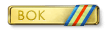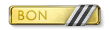# =FEW=Revolves

Members

9

## Community Reputation

1 Neutral

• Rank
Member
•1.## so i just created a computer language which just uses 10 assembly commands and can do anything a computer can

Still, I think I can say the above with confidence. Simply because it's not something the open source community considers as "We can solve it in the next 100 years" but it instead is of the category "Almost certainly impossible". Of course, lacking a proof renders this into speculation.
2.## so i just created a computer language which just uses 10 assembly commands and can do anything a computer can

The quantum computing thing: That's a common misconception. Quantum computers don't simulate all possible 2^n states using n qbits. If that were the case, then every single NP problem could be solved using a simple polynomial time and space algorithm using qbits. If this were actually the case, I could tell you a whole lot more of people would be researching quantum computing, not to mention a whole lot more money would be going into it right now. As it stands, only very few problems are actually known to be quickly solveable by quantum computers which classical computers can not do - in particular, integer factorization. See this comic (very scientific source ayyy lmao - but it truely is a concise description): http://www.smbc-comics.com/comic/the-talk-3
3. From my perspective I got hit by a short burst (all in the span of a second) - I didn't even see any tracers at all. Not doubting what happened on your screen - just super lag problems ;( The server crashed about 30 mins after you hit me.
4. It was my pleasure, always love to talk about the history/philosophy of math I can't speak for the physicists - some their statements irk me as well (I've studied math - not physics)
5. Yes the incompleteness theorem also applies to consitency of proofs using infinity. The theorem holds for mainstream mathematics as well as for the finite sort. IIRC you can prove the consistency of finite systems using an infinite one. Galileo's Paradox: The issue here is that we're fumbling about with what we mean by "size". The defination of size in math actually depends on the kind of object you're looking at, and how you're looking at it. There are several ways we can look at a subset of natural numbers: 1. Just as a set. This is Galileo's approach. 2. The algebraic way of looking at a set of natural numbers. (consider the natural numbers as a semiring - meaning you can add and multiple numbers together to get new ones - and sometimes subtract and divide) 3. The metric space of looking at a set of natural numbers (using the distance function d(n,m) = |n -m| you can tell the distance between any two numbers) (And probably way many more...) If we look at the set of natural numbers {0, 1, 2, 3, 4, 5, 6, 7, ...} using the different approches you'll see what I mean: Approach 1 (Just as a set) Since we see {0, 1, 2, 3, 4, 5, 6, 7, ...} merely as a box holding a bunch of stuff, we can rename it to {cheese, apple, fruit, banana, il-2, t-70, ...} and we wouldn't know the difference. That's why it's also ok to rename {0, 1, 2, 3, 4, 5, 6, 7, ...} to {0, 1, 4, 9, 16, ...}. That's why the two objects are the same. Approach 2 (The numbers are a semiring) Now we see {0, 1, 2, 3, 4, 5, 6, 7, ...} as a set where you can add two numbers (and multiply them) together, and get back a number in the set. For example, 1+2 =3 - we know that 3 is in the set. Note we can't replace the numbers with random words like we did in approach 1, since you can't add words together. We can not replace {0, 1, 2, 3, 4, 5, 6, 7, ...} with {0, 1, 4, 9, 16, ...} since the second object is NOT a semiring. This is because 1 + 4 = 5 - and 5 is not in the second set even though 1 and 4 are. So we see that the first object is strictly bigger than the second. Our defination of size has now changed - you can see it has something to do with addition and multiplication - instead of just renaming all the numbers without regards as to how they add and multiply. Approach 3 (The numbers are a metric space) Taking a metric space approach to the set {0, 1, 2, 3, 4, 5, 6, 7, ...} means we now consider the fact that we can tell the distance between two numbers. We can for example, now say that the distance between 3 and 5 is 2. Taking the set {0, 1, 4, 9, 16, ...} you can easily see that the distances are still defined. However, the two objects are NOT the same, because there are no two elements in {0, 1, 4, 9, 16, ...} which have the distance 2. But 1 and 3have the distance 2 in {0, 1, 2, 3, 4, 5, 6, 7, ...} . In fact, it's easy to show using this approach to size that {0, 1, 4, 9, 16, ...} is strictly smaller than {0, 1, 2, 3, 4, 5, 6, 7, ...} A bit of a mindfuck though: {0, 1, 2, 3, 4, 5, 6, 7, ...} has the same size as {0, 1, 4, 6, 8, 9, 10, 12 ...} (Remove all the primes) according to this defination of size. This is relatively easy to show. Conclusion: So if you really want to compare the size of two mathematical objects, you have to consider in what framework you're considering them in. Most people consider the natural numbers to be an algebraic structure - i.e. a semiring (even though most ppl don't know the terminology - just the ideas behind it). So when you bamboozle ppl about size using approach 1, you're really just stripping the numbers of what makes the numbers important. What is a 2 if I can't even do 2 + 2? Absolutely worthless. So why should I even bother to consider the 2 without being able to add it with other numbers? This should also apply to when I'm determining the size of a set of natural numbers.